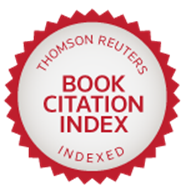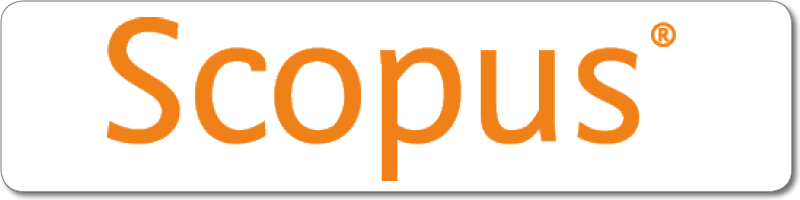| |
River Publishers Series in Mathematical, Statistical and Computational Modelling for Engineering

## Solid Geometry with MATLAB Programming

Authors:
Nita H. Shah, Gujarat University, India
Falguni S. Acharya, Parul University, India

ISBN: 9788770227612
e-ISBN: 9788770227605

Price: \$ 126.50Distributed exclusively by Routledge (Taylor & Francis Group)Description:

Solid geometry is defined as the study of the geometry of three-dimensional solid figures in Euclidean space. There are numerous techniques in solid geometry, mainly analytic geometry and methods using vectors, since they use linear equations and matrix algebra. Solid geometry is quite useful in everyday life, for example, to design different signs and symbols such as octagon shape stop signs, to indicate traffic rules, to design different 3D objects like cubicles in gaming zones, innovative lifts, creative 3D interiors, and to design 3D computer graphics. Studying solid geometry helps students to improve visualization and increase logical thinking and creativity since it is applicable everywhere in day-to-day life. It builds up a foundation for advanced levels of mathematical studies. Numerous competitive exams include solid geometry since its foundation is required to study other branches like civil engineering, mechanical engineering, computer science engineering, architecture, etc.

This book is designed especially for students of all levels, and can serve as a fundamental resource for advanced level studies not only in mathematics but also in various fields like engineering, interior design, architecture, etc. It includes theoretical aspects as well as numerous solved examples. The book includes numerical problems and problems of construction as well as practical problems as an application of the respective topic. A special feature of this book is that it includes solved examples using the mathematical tool MATLAB.

Keywords:

Plane, straight line, sphere, cone, cylinder, MATLAB programming